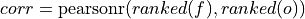# climpred.metrics._spearman_r#

climpred.metrics._spearman_r(forecast: xarray.Dataset, verif: xarray.Dataset, dim: Optional[Union[str, List[str]]] = None, **metric_kwargs: Any) [source]#

Spearman’s rank correlation coefficient.This correlation coefficient is nonparametric and assesses how well the relationship between the forecast and verification data can be described using a monotonic function. It is computed by first ranking the forecasts and verification data, and then correlating those ranks using the _pearson_r() correlation.

This is also known as the anomaly correlation coefficient (ACC) when comparing anomalies, although the Pearson product-moment correlation coefficient _pearson_r() is typically used when computing the ACC.

Note

Use metric _spearman_r_p_value() or _spearman_r_eff_p_value() to get the corresponding p value.

Parameters

Notes

 minimum -1.0 maximum 1.0 perfect 1.0 orientation positive

Example

>>> HindcastEnsemble.verify(
...     metric="spearman_r",
...     comparison="e2o",
...     alignment="same_verifs",
...     dim="init",
... )
<xarray.Dataset>
Coordinates:
* lead     (lead) int32 1 2 3 4 5 6 7 8 9 10
skill    <U11 'initialized'
Data variables:
SST      (lead) float64 0.9336 0.9311 0.9293 0.9474 ... 0.9465 0.9346 0.9328
Attributes:
`